### SOLUTIONS TO INTEGRATION OF EXPONENTIAL FUNCTIONS

SOLUTION 10 : Integrate. Use u-substitution. Let

u = 1+3e-x

so that (Don't forget to use the chain rule on e-x.)

du = 3e-x(-1) dx = -3e-x dx ,

or

(-1/3)du = e-x dx .

However, how can we replace the term e-3x in the original problem ? Note that.

From the u-substitution

u = 1+3e-x ,

we can "back substitute" with

e-x = (1/3)(u-1) .

Substitute into the original problem, replacing all forms of x, getting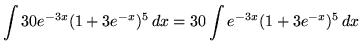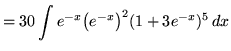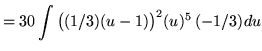(Recall that (AB)C = AC BC .)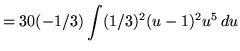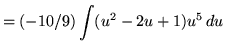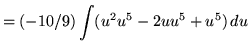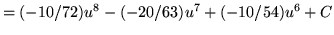.

SOLUTION 11 : Integrate. Use u-substitution. Let

u = e2x+6ex+ 1

so that (Don't forget to use the chain rule on e2x.)

du = (2e2x+6ex) dx

= (2ex+x+6ex) dx

= (2exex+6ex) dx

= 2ex(ex+3) dx

= 2ex(3+ex) dx

or

(1/2) du = ex(3+ex) dx .

Substitute into the original problem, replacing all forms of x, getting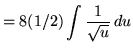(Do not make the following VERY COMMON MISTAKE :. Why is this INCORRECT ?)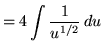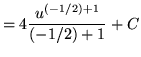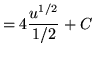.

SOLUTION 12 : Integrate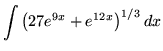. First, factor out e9x from inside the parantheses. Then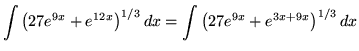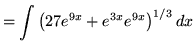(Recall that (AB)C = AC BC .)(Recall that (AB)C = ABC .)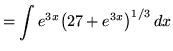.

Now use u-substitution. Let

u = 27+e3x

so that (Don't forget to use the chain rule on e3x.)

du = 3e3x dx ,

or

(1/3) du = e3x dx .

Substitute into the original problem, replacing all forms of x , and getting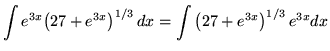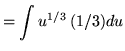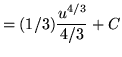.